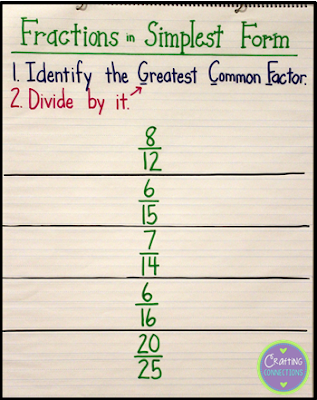Fractions in Simplest Form... An Interactive Anchor Chart | Crafting Connections

# Fractions in Simplest Form... An Interactive Anchor Chart

Today, I am sharing my "Fractions in Simplest Form" anchor chart with you. As you can see, this is an interactive anchor chart in which I create the basic shell prior to math class, but the five example problems are worked out in the presence of my students (and with their help).This shows how much of the anchor chart I create prior to class.

I model one row at a time, first enlisting students to help me list the factors for the numerator and the denominator, and then circling the greatest common factor.
Next, we divide both the numerator and denominators by the GCF and we have written our fraction in simplest form!

When we have finished working through the fractions on the anchor chart, it looks like this:

If you are looking for fraction resources, check out my store where I have created teaching fraction resources for 3rd grade, 4th grade, and 5th grade.  The introduction to calculating simplest form can be found in my fourth grade materials.

#### 1 comment:

1.I have been searching everywhere for something easy to use for my daughter. Thank you, this is perfect!